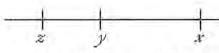If x > y and y > z, which of the follow...
If x > y and y > z, which of the following represents the greatest number?
• a)
x-z
• b)
x-y
• c)
y-x
• d)
z-y
• e)
z-x
If x > y and y > z, which of the following represents the greate...
From x > y and y > z, it follows that x > z.
These inequalities imply the following about the differences that are given in the answer choices:
Since the expressions in A and B represent positive numbers and the expressions in C, D, and E represent negative numbers, the latter can be eliminated because every negative number is less than every positive number. To determine which of x - z and x - y is greater, consider die placement of points widi coordinates x, y, and x on the number line,The distance between x and z (that is, x  - z) is the sum of the distance between x andy (that is, x - y) and the distance between y and z (that is, y - z).
Therefore (x- z) > (x y),which means that x-z represents the greater of the numbers represented by (x - z) and (x - y). Thus, x-z represents the greatest of the numbers represented by&e answer choices.
Alternatively,
y > z given
-y < -z multiply both sides by -1
x - y < x - z add x to both sides
Thus, x - z represents the greater of the numbers represented by (x - z) and (x - y). Therefore, x - z represents the greatest of the numbers represented by the answer choices.
 1 Crore+ students have signed up on EduRev. Have you?
1 Crore+ students have signed up on EduRev. Have you?

### Learn this topic in detailGMAT Mock Test - 1 79 Ques | 158 MinsMore from Related Course Quantitative for GMATView courses related to this question

### Quick links for GMAT exam850+
Video Lectures2500+
Revision Notes600+
Online Tests10,000+
Doubts Solved
If x > y and y > z, which of the following represents the greatest number?a)x-zb)x-yc)y-xd)z-ye)z-xCorrect answer is option 'A'. Can you explain this answer?
Question Description
If x > y and y > z, which of the following represents the greatest number?a)x-zb)x-yc)y-xd)z-ye)z-xCorrect answer is option 'A'. Can you explain this answer? for GMAT 2023 is part of GMAT preparation. The Question and answers have been prepared according to the GMAT exam syllabus. Information about If x > y and y > z, which of the following represents the greatest number?a)x-zb)x-yc)y-xd)z-ye)z-xCorrect answer is option 'A'. Can you explain this answer? covers all topics & solutions for GMAT 2023 Exam. Find important definitions, questions, meanings, examples, exercises and tests below for If x > y and y > z, which of the following represents the greatest number?a)x-zb)x-yc)y-xd)z-ye)z-xCorrect answer is option 'A'. Can you explain this answer?.
Solutions for If x > y and y > z, which of the following represents the greatest number?a)x-zb)x-yc)y-xd)z-ye)z-xCorrect answer is option 'A'. Can you explain this answer? in English & in Hindi are available as part of our courses for GMAT. Download more important topics, notes, lectures and mock test series for GMAT Exam by signing up for free.
Here you can find the meaning of If x > y and y > z, which of the following represents the greatest number?a)x-zb)x-yc)y-xd)z-ye)z-xCorrect answer is option 'A'. Can you explain this answer? defined & explained in the simplest way possible. Besides giving the explanation of If x > y and y > z, which of the following represents the greatest number?a)x-zb)x-yc)y-xd)z-ye)z-xCorrect answer is option 'A'. Can you explain this answer?, a detailed solution for If x > y and y > z, which of the following represents the greatest number?a)x-zb)x-yc)y-xd)z-ye)z-xCorrect answer is option 'A'. Can you explain this answer? has been provided alongside types of If x > y and y > z, which of the following represents the greatest number?a)x-zb)x-yc)y-xd)z-ye)z-xCorrect answer is option 'A'. Can you explain this answer? theory, EduRev gives you an ample number of questions to practice If x > y and y > z, which of the following represents the greatest number?a)x-zb)x-yc)y-xd)z-ye)z-xCorrect answer is option 'A'. Can you explain this answer? tests, examples and also practice GMAT tests.(Scan QR code)